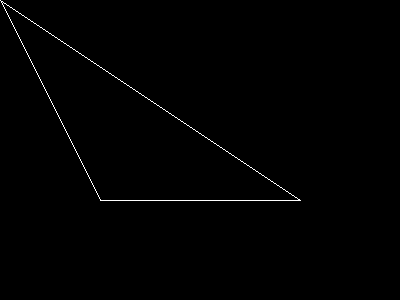# imagepolygon

(PHP 4, PHP 5)

imagepolygon βÄî Draws a polygon

### Description

bool imagepolygon ( resource \$image , array \$points , int \$num_points , int \$color )

imagepolygon() creates a polygon in the given image .

### Parameters

image

An image resource, returned by one of the image creation functions, such as imagecreatetruecolor().

points

An array containing the polygon's vertices, i.e. points = x0, points = y0, points = x1, points = y1, etc.

num_points

Total number of points (vertices)

color

A color identifier created with imagecolorallocate()

### Return Values

Returns TRUE on success or FALSE on failure.

### Examples

Example#1 imagepolygon() example

``` <?php// create a blank image\$image = imagecreatetruecolor(400, 300);// fill the background color\$bg = imagecolorallocate(\$image, 0, 0, 0);// choose a color for the polygon\$col_poly = imagecolorallocate(\$image, 255, 255, 255);// draw the polygonimagepolygon(\$image, array (        0,   0,        100, 200,        300, 200    ),    3,    \$col_poly);// output the pictureheader("Content-type: image/png");imagepng(\$image);?> ```

The above example will output something similar to: